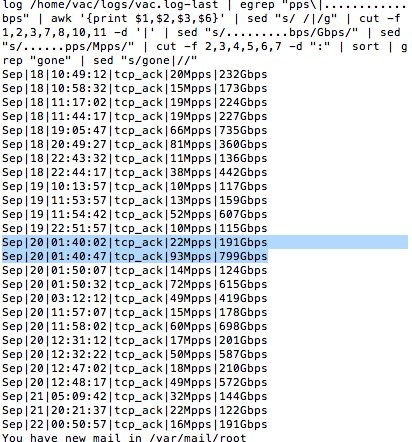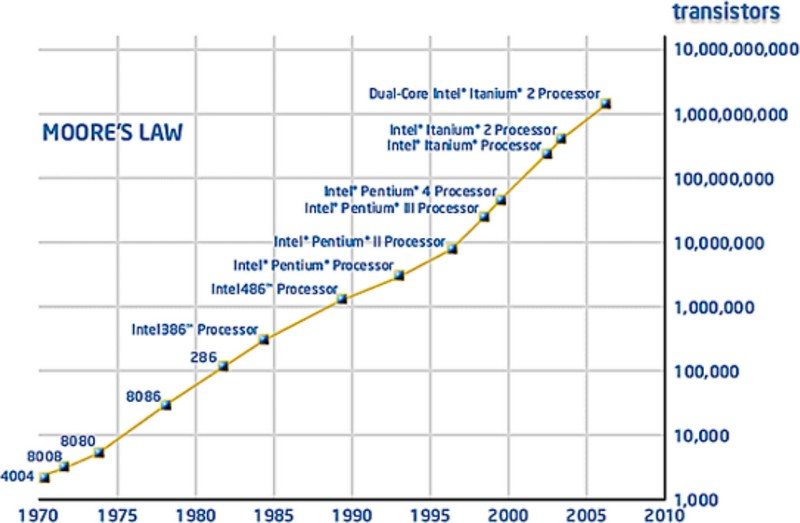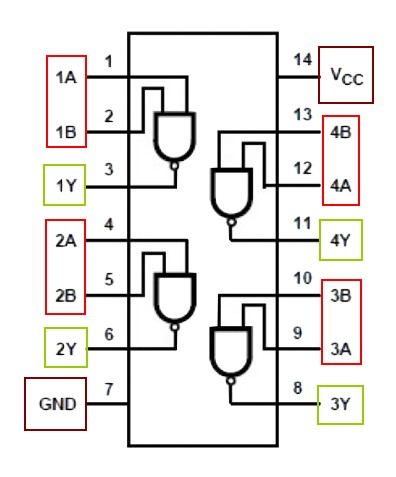# Boolean - (Logical) Operator (OR, AND, XOR, )

Boolean operator manipultes truthy and falsy values that can come from:

Boolean operator has the same than arithmetic bitewise operator

## Syntax

General syntax is

````lhs operator rhs`
```

where:

### Negation

Any Boolean expression (such as the outcome of a comparison operator may be negated with not.

Syntax example:

``````not(expression)
# or
!(expression)```
```

### Operator

Operator Description Logic
&& Logical AND If rhs is falsy, the expression evaluates to lhs
|| Logical OR if lhs is truthy, the expression evaluates to lhs, otherwise, it evaluates to rhs
& Bitwise AND
| Bitwise OR
^ Bitwise exclusive OR

&& and || exhibit short-circuiting“ behavior, which means that the second operand is evaluated only if needed

## Computer

In computer, a logical operator is implemented by a logic gate

## Order of precedence

And and OR have normally lower priorities than comparison operators

Between them, not has the highest priority and or the lowest, so that:

````A and not B or C`
```

is equivalent to

````(A and (not B)) or C`
```

.

## Illustration

And, Or and Xor seem incorrect.

Recommended PagesBash - Boolean Operator

This page is Boolean operator in bash. operators Symbol Logic && || ! not Details: True if expression is false. True if both expression1 and expression2 are true. True if either...Bit - Bitwise and Shift binary operator

Operator on bit. See Reference/Operators/Bitwise_Operators assignment operator the bitwise arithmetic operators (~, &, ^, and |) and the shift operators (<<, >>, and >>>). The bitwise...Boolean - Predicate (Boolean Expression, Condition)

A Predicate is an boolean expression that evaluates to: TRUE, FALSE, or UNKNOWN. A predicate acts as a filter that filters a certain number of element from a collection (set) where: ...Computer Architecture - Arithmetic logic unit (ALU)

The ALU is a component of a CPU. It is a device that executes all arithmetic and logical operations. In most arithmetic logic unit (ALU) hardware, we find a fixed-precision arithmetic found , which...Computer System

A computer is a device of device controlled by a special device called a CPU. It consists then of: at least a processor (CPU), a memory device where: primary storage holds data only temporarily,...Device - Logic Gate (Nand, And, Or, Xor, )

A Logic Gate is a device that: implements a boolean function (true or false) and therefore implement binary operations. It has: two binary inputs with the value on or off (ie a bit) one binary...Instruction - Execution (Execution environment)

A execution environment is an environment that provides the facility to make instruction executable. It's a sort of context at the computer device level. Execution is the third step in a instruction...
Javascript - Logical Operator

Logical operator in Javascript &&: AND Returns expr1 if it can be converted to true; otherwise, returns expr2. See Usage Example: Initialization even if it's a null object Snippet example:...Language - Operator

An operator is a symbol (token) that compose an expression. It operates on values on its side (left and/or right). They represents a function but where the argument are on both side of it. This...Ordinal Data - Comparison Operator (Order, Equality)

A comparison operator is a class of operators that model: a order or a equivalence relationship A comparison operator test the order relationship between two operands and returns a Boolean. The...